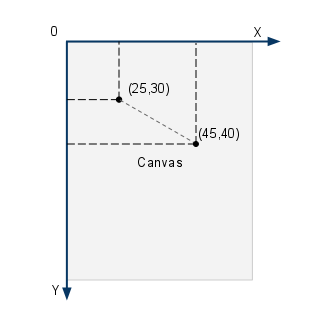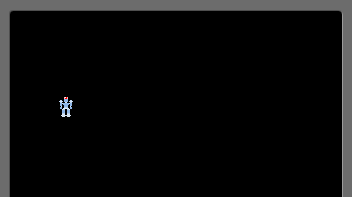# Moving Images on the Screen with Android

In the previous post we've displayed an image and implemented a very simple drag functionality. Things we should know by now following the series:

• launch an Android application in full screen

• use a separate thread which controls the application (the game loop)

• load an image from the resources

• acquire the canvas and draw the image onto it

• handle basic touch gestures

The task I'm setting for this entry is simple: have the droid travel through the screen. It should never leave the surface and it should bounce back when it hits the wall which is the edge of the screen.

If you remember the image is just a representation of the droid.

So we will modify the droid object and we'll add some abilities.

Just one for the time being. Our droid is movable. It can move. This implies that it has speed. We will vest it with the ability of movement. To achieve this we will add a `move()` method and this method will just update the `X` and `Y` coordinates based on its `Speed`. `Speed` will be a class in itself and the Droid will contain it. I will do a concrete implementation now but later on I will be using the Strategy Pattern.

Create the `Speed.java` class.

``````
package net.obviam.droidz.model.components;

public class Speed {

public static final int DIRECTION_RIGHT = 1;

public static final int DIRECTION_LEFT = -1;

public static final int DIRECTION_UP = -1;

public static final int DIRECTION_DOWN = 1;

private float xv = 1; // velocity value on the X axis

private float yv = 1; // velocity value on the Y axis

private int xDirection = DIRECTION_RIGHT;

private int yDirection = DIRECTION_DOWN;

public Speed() {

this.xv = 1;

this.yv = 1;

}

public Speed(float xv, float yv) {

this.xv = xv;

this.yv = yv;

}

public float getXv() {

return xv;

}

public void setXv(float xv) {

this.xv = xv;

}

public float getYv() {

return yv;

}

public void setYv(float yv) {

this.yv = yv;

}

public int getxDirection() {

return xDirection;

}

public void setxDirection(int xDirection) {

this.xDirection = xDirection;

}

public int getyDirection() {

return yDirection;

}

public void setyDirection(int yDirection) {

this.yDirection = yDirection;

}

// changes the direction on the X axis

public void toggleXDirection() {

xDirection = xDirection * -1;

}

// changes the direction on the Y axis

public void toggleYDirection() {

yDirection = yDirection * -1;

}

}

``````

We'll use direction constants to determine the movement direction on the axis.

The droid has a vertical and a horizontal speed and at each game update the coordinates are set considering the direction of the movement.

The droid will be allowed to move only on the area of the canvas. That is a rectangle and our 2D coordinate system. Unlike in the math classes the origin is in the top left corner. So for the droid to start from the top left corner of the screen its coordinates will be `0,0`. To move in a diagonal line the speed will be `1` for both the `X` and `Y` components of the speed vector. To move towards the bottom right the directions will be: `1 (right)` for the `X` axis and `1 (down)` for the `Y` axis.Canvas Coordinate System

To have the droid move horizontally the speed of the `Y` vector must be `0`. A value of `0.5` for `Y` and `1` for `X` will make the droid travel at a `22.5` degrees to the `X` axis. Simple geometry.

In the `Speed` we have the vector components (`x` and `y`) and the directions along with the getters and setters. The two methods (`toggleXDirection()` and `toggleYDirection()` just change the direction with one call. We'll see later at collision detection (with the wall of the screen) that it is pretty useful.

The game loop (`MainThread.java`) gets an important modification as it gets the game update method introduced. The following code snippet is the updated `run()` method which has just one line added:

``````
this.gamePanel.update();

``````

The `run()` method:

``````
public void run() {

Canvas canvas;

Log.d(TAG, "Starting game loop");

while (running) {

canvas = null;

// try locking the canvas for exclusive pixel editing

// in the surface

try {

canvas = this.surfaceHolder.lockCanvas();

synchronized (surfaceHolder) {

// update game state

this.gamePanel.update();

// render state to the screen

// draws the canvas on the panel

this.gamePanel.render(canvas);

}

} finally {

// in case of an exception the surface is not left in

// an inconsistent state

if (canvas != null) {

surfaceHolder.unlockCanvasAndPost(canvas);

}

} // end finally

}

}

``````

We will create the corresponding method in the `MainGamePanel`. This method is in charge of updating the state of all the objects in the application. Currently only the droid. Because the droid is moving we will introduce a basic collision detection with the walls. The logic is simple. Check if the droid is moving towards left then check if the droid's position is at the wall and if it is then change its direction. Bear in mind that the droid's position is the center of the image so we need to use the image's width and height to get the accuracy right.

We also update the position of the droid. To keep the update method simple we delegate the update of the droid's position to the droid itself. So the droid will get an update method which will keep updating its position if the droid is not being picked up by a touch gesture. Check the previous post for this.

Check the code:

`MainGamePanel.java`

``````
public void update() {

// check collision with right wall if heading right

if (droid.getSpeed().getxDirection() == Speed.DIRECTION_RIGHT

&& droid.getX() + droid.getBitmap().getWidth() / 2 >= getWidth()) {

droid.getSpeed().toggleXDirection();

}

// check collision with left wall if heading left

if (droid.getSpeed().getxDirection() == Speed.DIRECTION_LEFT

&& droid.getX() - droid.getBitmap().getWidth() / 2 <= 0) {

droid.getSpeed().toggleXDirection();

}

// check collision with bottom wall if heading down

if (droid.getSpeed().getyDirection() == Speed.DIRECTION_DOWN

&& droid.getY() + droid.getBitmap().getHeight() / 2 >= getHeight()) {

droid.getSpeed().toggleYDirection();

}

// check collision with top wall if heading up

if (droid.getSpeed().getyDirection() == Speed.DIRECTION_UP

&& droid.getY() - droid.getBitmap().getHeight() / 2 <= 0) {

droid.getSpeed().toggleYDirection();

}

// Update the lone droid

droid.update();

}

``````

`getWidth()` and `getHeight()` return the width and height of the view. The panel is a view, remember?

The `Droid.java` file's `update()` method:

``````
public void update() {

if (!touched) {

x += (speed.getXv() * speed.getxDirection());

y += (speed.getYv() * speed.getyDirection());

}

}

``````

I also changed the render's name in the `MainThread.java` so now it is render instead if `onDraw`. Just that I like it better as it follows the `update` -> `render` naming.

Run the application and you should see a screen like the following one with the droid moving in a 45 degrees angle and bouncing off the walls as it hits them. You can also drag the droid around.

To exit the application click (touch) the lower part of the screen.Moving Droid